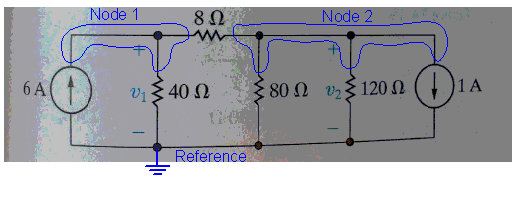# Use the node-voltage to find v1 and v2 in the circuit below

Firben
Use the node-voltage to find v1 and v2 in the circuit below(see picture)

4.8)
http://s716.photobucket.com/albums/ww168/Pitoraq/?action=view&current=DSC_0204.jpg

http://s716.photobucket.com/albums/ww168/Pitoraq/?action=view&current=DSC_0206.jpg

4.26)

http://s716.photobucket.com/albums/ww168/Pitoraq/?action=view&current=DSC_0205.jpg

http://s716.photobucket.com/albums/ww168/Pitoraq/?action=view&current=DSC_0207.jpg

## Homework Equations

4.8)

v1 + (v1-v2)/8 = 6 (node 1)
1 + v2/120 = (v2-v1)/8 (node 2)

4.26

1/R(eq) = 1/150 + 1/75 = 30 ohm

2 - ((-v1-25)/50) + v1/150 + v1/75 = 0

## The Attempt at a Solution

4.8)
After combining node 1 and node 2 i got v = 146 v which is wrong

what about the r=80 resistor ?

4.26)

I got v1 to be -37.5 v and p = (-37.5)*2 = -75 W

a) -37.5 V,75W
b) -37.5 v, 75W

## The Attempt at a Solution

Last edited:

Mentor

Check carefully the implied direction of the currents in your Node 2 equation.

Firben

But i can't see anything wrong with the current direction

Mentor

1 + v2/120 = (v2-v1)/8 (node 2)

On the left you have currents flowing out of node 2. On the right you also have a current flowing out of node 2. If it's on the right, and it must balance the currents on the left, it should be flowing INTO node two.

You might find it more straightforward to write the sum of all currents on one side, setting the sum to zero. That way the individual current directions may be more obvious.

One other thing: what happened to the contribution of the 80 Ohm resistor?

Firben

Yes. I started with writing
1 + v2/120 - (v2-v1)/8 = 0
But I am not sure about the 80 ohm resistor

Mentor

Yes. I started with writing
1 + v2/120 - (v2-v1)/8 = 0
Okay, but since you are summing all the currents LEAVING the node, the sign on the last term should be positive, too.
But I am not sure about the 80 ohm resistor

Add a term for it that is just like the one for the 120 Ohm resistor.

Firben
From the first node equation, should the 80 resistor be added to that to ?

Firben

Okay, but since you are summing all the currents LEAVING the node, the sign on the last term should be positive, too.

I have done that:

1 + v2/120 -(v2-v1)/8 = 0

1 + v2/120 leaves the node 2, while (v2-v1)/8 enters node 2.

Should i add the resistors: 1/R = 1/80 + 1/120 , R = 48 ohm ?

-6 + v1/40 + (v1-v2)/8 = 0 (node 1)
1 + v2/48 - (v2-v1)/8 = 0 (node 2)

Are the equations right ?

Last edited:
Mentor
From the first node equation, should the 80 resistor be added to that to ?

Nope. The 80Ω path "belongs" to the second node; it directly connects to the second node, whereas there is the 8Ω resistor between it and the first node.Each path through which current can flow into (or out of) a given node needs to have a term in the node's equation.

Out of habit I tend to assume that all currents, except explicitly defined ones such as fixed current sources, always flow out of a given node, and just bang down the equation accordingly all on the left hand side. The math will automatically take care of the details of the "true" current directions since its the relative potentials that define them anyways, and they start out as unknowns.

So, can you now take another stab at writing the two node equations?

#### Attachments

Firben
I got it to be:
-6 + v1/40 + (v1-v2)/8 = 0 (node 1)
1 + v2/120 - v2/80 - (v2-v1)/8 = 0 (node 2)
Is this correct ?

Mentor
I got it to be:
-6 + v1/40 + (v1-v2)/8 = 0 (node 1)
1 + v2/120 - v2/80 - (v2-v1)/8 = 0 (node 2)
Is this correct ?

The node 1 equation looks fine.

You've got some sign issues with the node 2 equation. For example, why would the signs for the 80Ω and 120Ω paths be different? Surely current must flow in the same direction through the two?

Firben
The node 1 equation looks fine.

You've got some sign issues with the node 2 equation. For example, why would the signs for the 80Ω and 120Ω paths be different? Surely current must flow in the same direction through the two?

Then it must be:

1 + v2/120 + v2/80 -(v2-v1)/8 = 0

Should 80 ohm be possitive ?

Mentor
Then it must be:

1 + v2/120 + v2/80 -(v2-v1)/8 = 0

Should 80 ohm be possitive ?

ALL of the terms should be positive if you're summing them as outgoing currents.

Firben
ALL of the terms should be positive if you're summing them as outgoing currents.

Shouldn't (v2-v1)/8 be negative since 6A goes into node 2 ? Furthermore shouldn't the sum of all be zero ?

Mentor
Shouldn't (v2-v1)/8 be negative since 6A goes into node 2 ? Furthermore shouldn't the sum of all be zero ?

Currently the potentials V1 and V2 are unknowns. Regardless of whether you know by intuition that current will flow from the V1 node to the V2 node, you can rely on the math to take care of that detail. By writing that current as (V2 - V1)/8, it will automatically evolve to have V1 > V2, so that the term's actual sign will take care of itself. This means that you don't have to rely on intuition when a complex circuit doesn't make it obvious.

So, if you always write the expressions assuming that the current node has a higher potential than the surrounding ones, and that all currents are outgoing (or incoming --- your choice), then the math will take care of the rest. Less thinking = fewer missteps.